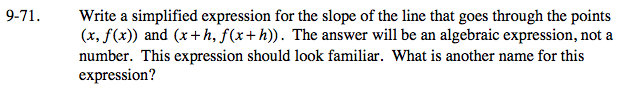### Home > AC > Chapter 16 > Lesson 16.9.2.1 > Problem9-71

9-71.

Write a simplified expression for the slope of the line that goes through the points (x, f(x)) and (x + h, f(x + h)). The answer will be an algebraic expression, not a number. This expression should look familiar. What is another name for this expression? Homework Help ✎$\frac{f(x+h)-f(x)}{(x+h)-x}$